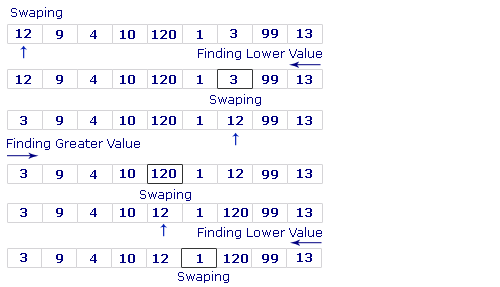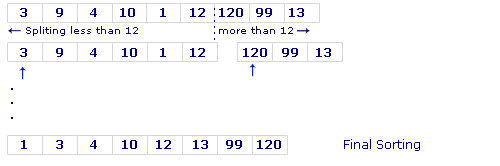Tutorials

# Quick Sort In Java

In this example we are going to sort integer values of an array using quick sort.

In this example we are going to sort integer values of an array using quick sort.

# Quick Sort in Java

Introduction

In this example we are going to sort integer values of an array using quick sort.

Quick sort algorithm is developed by C. A. R. Hoare. Quick sort is a comparison sort. The working of  quick sort algorithm is depending on a divide-and-conquer strategy. A divide and conquer strategy is  dividing  an array  into two sub-arrays. Quick sort is one of the fastest and simplest sorting algorithm. The complexity of quick sort in the average case is  Θ(n log(n)) and in the  worst case is Θ(n2).

Code description:

In quick sort algorithm pick an element from array of elements. This element is called the pivot. Then compare the the values from left to right until a greater element is find then swap the values. Again start comparison from right with pivot. When lesser element is find then swap the values. Follow the same steps until  all elements which are less than the pivot come before the pivot and all elements greater than the pivot come after it. After this partitioning, the pivot is in its last position. This is called the partition operation. Recursively sort the sub-array of lesser elements and the sub-array of greater elements.

Working of quick sort
algorithm:
Input:
12 9 4 99 120 1 3 10 13Output:
1 3 4 10 12 13 99 120

The code of the program :

 ``` public class QuickSort{   public static void main(String a[]){   int i;   int array[] = {12,9,4,99,120,1,3,10,13};   System.out.println("\n\n RoseIndia\n\n");   System.out.println(" Quick Sort\n\n");   System.out.println("Values Before the sort:\n");   for(i = 0; i < array.length; i++)   System.out.print( array[i]+"  ");   System.out.println();   quick_srt(array,0,array.length-1);   System.out.print("Values after the sort:\n");   for(i = 0; i = n) {   return;   }   int mid = array[(lo + hi) / 2];   while (lo < hi) {   while (lo mid) {   hi--;   }   if (lo < hi) {   int T = array[lo];   array[lo] = array[hi];   array[hi] = T;   }   }   if (hi < lo) {   int T = hi;   hi = lo;   lo = T;   }   quick_srt(array, low, lo);   quick_srt(array, lo == low ? lo+1 : lo, n);   } }```

Output of the example:

 `C:\array\sorting>javac QuickSort.java` ```C:\array\sorting>java QuickSort ``` ``` RoseIndia ``` ``` Quick Sort ``` `Values Before the sort:` ```12 9 4 99 120 1 3 10 13 Values after the sort: 1 3 4 9 10 12 13 99 120 PAUSE``` `C:\array\sorting>_`